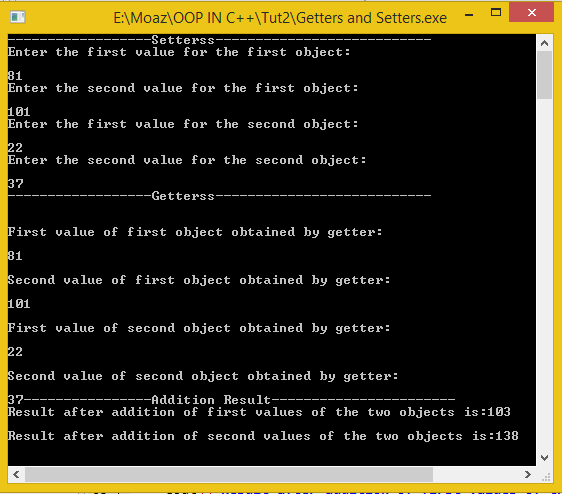# Setter and Getter functions in C++

In this part you will learn: 1. How to make an object of class in C++ 2. What are Setters 3. What are getters 4. To use setters and getters 5. Basic C++ syntax How to make an object of class in C++? Whenever we want to make an object of the class we first define its class and then in the main of the program we write name of the class followed by the name of the object we want to make. e.g to make an object we write the code as class anyclass{ //data members and functions }; int main { anyclass myobj; } In the above code we have created an object of the class ‘anyclass’ whose name is ‘myobj’ What are Setters? Specific functions which are used to set or assign the values to the data members of objects of some class are called setters. There could be any name of the function which set the value of data member but usually it is called setter. The code below in this tutorial will have setter which will make things more clearer. What are Getters? Getters are the functions that retrieve the values of data members of some object so that these values can be used in the main of the program. As in C++ each function can return only one variable so here we need to have the getters equal to number of the data members so that each getter returns the value of one data member. The code below in this tutorial will have getters which will make things more clearer. Program:
`#include<iostream>#include<conio.h>using namespace std;`
These are the basic header files which are required for C++ programming.
`class myFirstClass{private:  int num1;  int num2;public:  void setValue(int a,int b) //setter  {  num1=a;  num2=b;}`
In the above code we have made a class named ‘myFirstClass’, its private data members are num1 and num 2 which have type of integers. It also contains member functions which are under the public access specifier of the class. It should be noted here that data members are always kept private because an important thing kept in mind while working in object oriented programming is that it hides the implementation of the class by hiding the details(also called encapsulation of data) so otherwise class and structure will have no difference. In the next part we have the setter function named ‘setValue’ of the class that takes in two input arguments and assign each argument to the data member of the class hence setting the values. It must be noted here that the setters never return a value so we the return type of setters is always void(meaning empty).
`int getValue1(){  return num1;}int getValue2(){    return num2;} int addFirstNum(myFirstClass obj){  int num1Total;  num1Total=num1+obj.num1;  return num1Total;}int addSecondNum(myFirstClass obj){  int num2Total;  num2Total=num2+obj.num2;  return num2Total;}};`
In this part of code functions int getValue1() and int getValue2() are the getters, as already told before the getters are equal to the number of data members so here we have two getters and two data members as well. getValue1() returns the value of first data member ‘num1’ while the getValue2() function returns the value of second data member ‘num2 ’ and it must be noted here that as the both getters return the integer type values so there return type is int. In the last two function of the class ‘int addFirstNum(myfirstclass obj)’ and ‘int addSecondNum(myfirstclass obj)’we basically are adding the corresponding data members of the two objects. Here this must be kept in mind that the object through which the function is called(the object after which dot operator ‘.’is used) has the name of attributes same as defined in class while the object which is delivered to the function as argument uses dot operator to call its data member as shown in above example. The result of addition is stored in another integer type variable which is then returned.
`void main(){   myFirstClass obj1;  myFirstClass obj2;  int a,b,c,d;  cout<<"------------------Setterss---------------------------\n";  cout<<"Enter the first value for the first object:\n\n";  cin>>a;  cout<<"Enter the second value for the first object:\n\n";  cin>>b;  cout<<"Enter the first value for the second object:\n\n";  cin>>c;  cout<<"Enter the second value for the second object:\n\n";  cin>>d;   obj1.setValue(a,b);  obj2.setValue(c,d);  cout<<"------------------Getterss---------------------------\n";  cout<<"\n\nFirst value of first object obtained by getter:\n\n"<<obj1.getValue1();  cout<<"\n\nSecond value of first object obtained by getter:\n\n"<<obj1.getValue2();  cout<<"\n\nFirst value of second object obtained by getter:\n\n"<<obj2.getValue1();  cout<<"\n\nSecond value of second object obtained by getter:\n\n"<<obj2.getValue2();  //now for addition:  cout<<"----------------Addition Result-----------------------\n";  cout<<"Result after addition of first values of the two objects is:"<<obj1.addFirstNum(obj2);  cout<<"\n\nResult after addition of second values of the two objects is:"<<obj1.addSecondNum(obj2);  getch();}`
In the main of the function we first made two objects named ‘obj1’ and ‘obj2’ of the class ‘myFirstClass’ and in the next lines by using setValue function we are assigning the values to the data members of each object which are input by the user. Then we are displaying the result on screen by using the getValue(also called getter) functions. In the last part we are displaying the result after addition of the corresponding data members of the two objects for which we are using functions addFirstNumand addSecondNum. Here the object through which function is called always comes before the dot operator and after the dot operator the name of function is written, here the function is passed the obj2 so that their data members be added. Output: